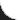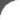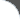Home | Tutorials | Articles | Videos | Products | Tools | Search
 Interviews | Open Source | Tag Cloud | Follow Us | Bookmark | ContactIn Browser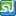StumbleUpon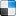del.icio.us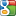Google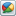Google Buzz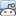reddit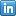LinkedIn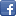Facebook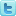TwitterLinkedin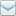E-Mail
 Tools and Libs > Uncommons Maths > Greatest Common Divisor

# Greatest Common Divisor

Uncommons Maths is a java library with nice math utilities and random number generators. This requires the library uncommons-maths-1.2.2.jar to be in classpath. The following example shows using Maths.greatestCommonDivisor() API.

 File Name  : com/bethecoder/tutorials/uncommons/GCDTest.java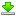Author  : Sudhakar KV Email  : [email protected]

 ``` package com.bethecoder.tutorials.uncommons; import org.uncommons.maths.Maths; public class GCDTest {   /**    * @param args    */   public static void main(String[] args) {          long a = 24;     long b = 32;     long c = Maths.greatestCommonDivisor(a, b);     System.out.println("GCD of (" + a + ", " + b + ") = " + c);          a = 1729;     b = 1331;     c = Maths.greatestCommonDivisor(a, b);     System.out.println("GCD of (" + a + ", " + b + ") = " + c);          a = 123456;     b = 4684;     c = Maths.greatestCommonDivisor(a, b);     System.out.println("GCD of (" + a + ", " + b + ") = " + c);   } }```

It gives the following output,
```GCD of (24, 32) = 8
GCD of (1729, 1331) = 1
GCD of (123456, 4684) = 4
```